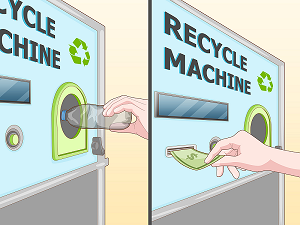### B - Bottles recycling

##### Languages: C, C++, Java, Python, ... (details)

Fito is a genius! He just invented something that will change the world: the best way to recycle bottles. The idea is that every time you buy a bottle you will be charged $y$ extra pesos on top of the original price $x$. Then, when you return the empty botle you will get the $y$ extra pesos back. To make the process faster every supermarket will have a machine that automatically takes the bottles and give you the extra money back. With that money, you could actually buy new bottles. Isn't it an amazing idea?Despite his great invention, we already know that Fito is not very good at programming. Therefore, as usual, he needs your help. He would like you to write a program that computes for a given amount of money $L$, assuming all bottles cost $x$ pesos, how many new bottles a person can buy, taking into account that the person can return the empty bottles to get the extra money back, then buy some new bottles, return the empty ones again and repeat this process until he/she does not have enough money to buy anything else.

#### Input

The only line of input will contain $3$ integer numbers: the total amount of money $L$ ($0 \leq L \leq 10^{18}$) the person has at the beginning, the cost of one bottle $x$ ($1 \leq x \leq 100$) and the extra money one has to pay per bottle $y$ ($0 \leq y < x$).

#### Output

Print one line with the maximum amount of bottles a person can buy in total having initially $L$ pesos.

#### Sample test(s)

Input
150 10 5
Output
14
Input
1000000000000000000 1 0
Output
1000000000000000000

#### Hints

In the first case, the person can buy $10$ bottles ($10 \times 15 = 150$ pesos). Then return the $10$ empty bottles and get $10 \times 5=50$ pesos back. With $50$ pesos the person can still buy $3$ more bottles ($3 \times 15 = 45$). After returning the $3$ empty bottles, he/she will have $5 + 3 \times 5 = 20$ and could buy another bottle. After returning it, there will be $5+5=10$ pesos which are not enough for buying more bottles. There is no other way in which a person could buy more than $14$ bottles. Therefore, the answer is $14$.

This recycling system is quite popular in Germany!